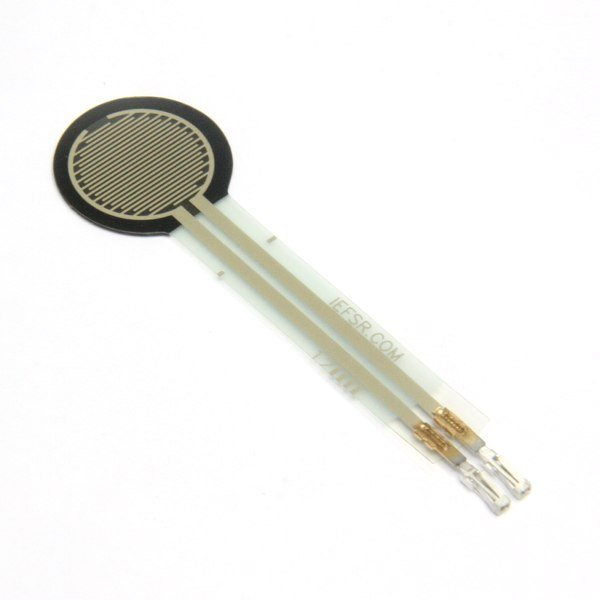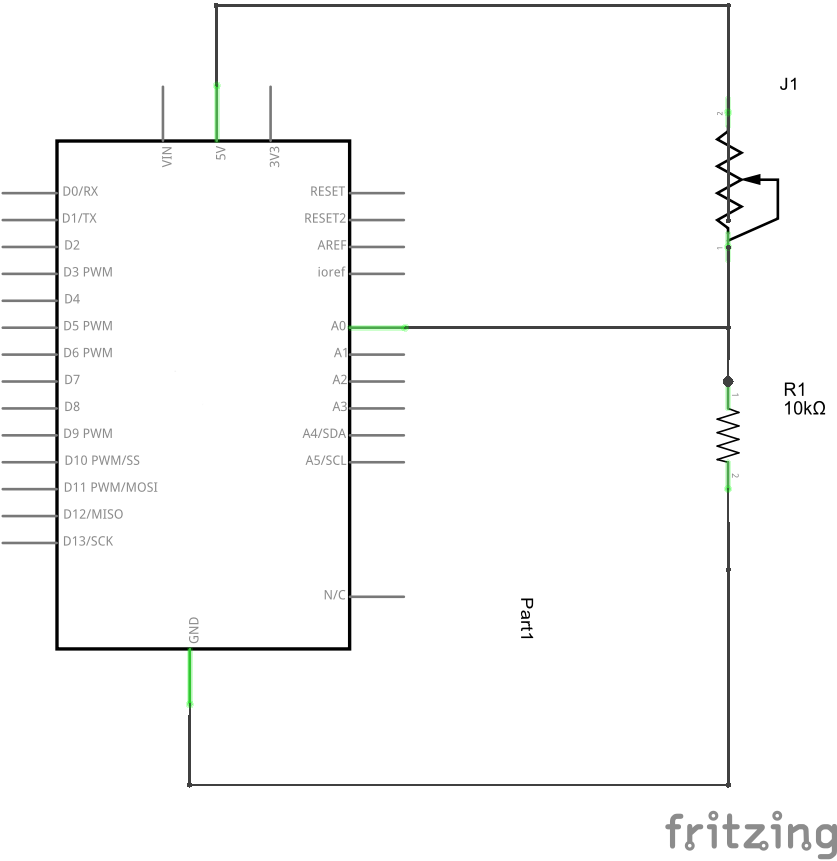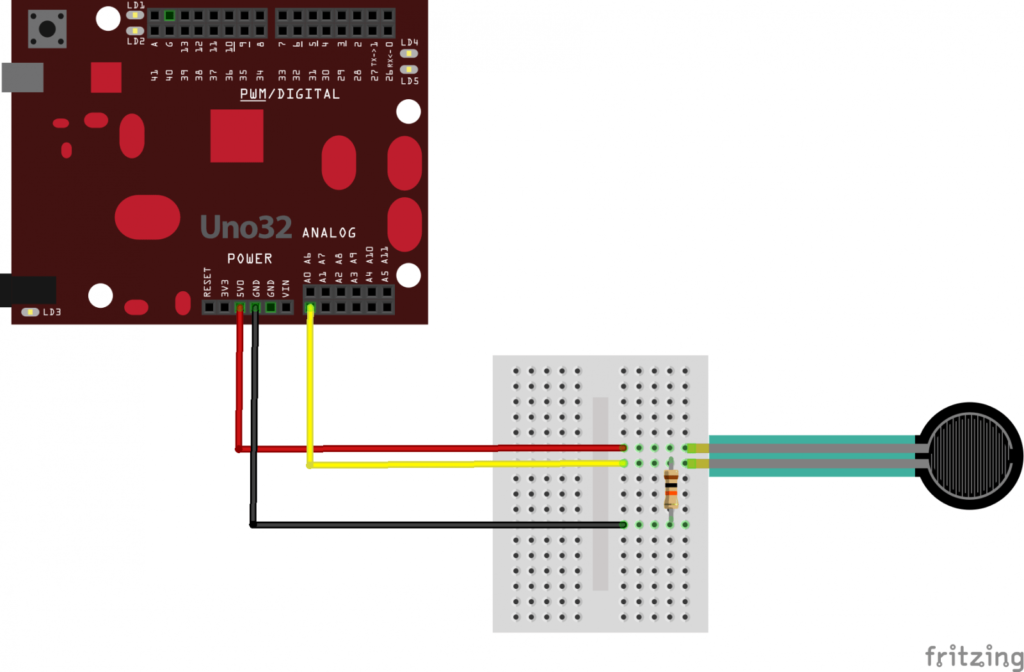Home Chipkit Chipkit Uno force sensitive resistor example

# Chipkit Uno force sensitive resistor example

A force-sensing resistor is a material whose resistance changes when a force or pressure is applied.

Force-sensing resistors consist of a conductive polymer, which changes resistance in a predictable manner following application of force to its surface.They are normally supplied as a polymer sheet or ink that can be applied by screen printing. The sensing film consists of both electrically conducting and non-conducting particles suspended in matrix. The particles are sub-micrometre sizes, and are formulated to reduce the temperature dependence, improve mechanical properties and increase surface durability. Applying a force to the surface of the sensing film causes particles to touch the conducting electrodes, changing the resistance of the film.

As with all resistive based sensors, force-sensing resistors require a relatively simple interface and can operate satisfactorily in moderately hostile environments. Compared to other force sensors, the advantages of FSRs are their size (thickness typically less than 0.5 mm), low cost and good shock resistance. A disadvantage is their low precision: measurement results may differ 10% and more

Here is a typical exampleNow we will show you how to connect this to your Chipkit Uno

Schematic

A simple schematic to build here basically you are creating a  voltage divider with 10k resistor and the force sensor, squeezing the force sensor alters the resistance so the voltage in at A0 will vary depending on the forceHere is a layout which you could build on a breadboardCode

Pretty simple example, the analog input is echoed via the serial monitor

[codesyntax lang=”cpp”]

```int forcePin = 0;

void setup(void)
{
Serial.begin(9600);   // We'll send debugging information via the Serial monitor
}

void loop(void)
{

delay(500);
}```

[/codesyntax]

Results

Open the serial monitor and then squeeze the sensor, you should see something like the following